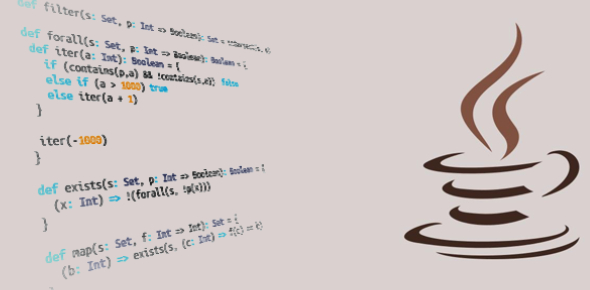# Basics Of Java Programming Language: Quiz!

13 Questions | Total Attempts: 2403Settings.

• 1.
Before you start: if something is written in quotation marks("quote") then it means Java code What is the name for an application that changes a human-readable programming language into a machine-readable language?
• A.

Compiler

• B.

Converter

• C.

Encoder

• 2.
Which applications can you use to edit Java code?
• A.

Any Text editor

• B.

Software development environments (Eclipse, IDE)

• C.

Graphic Editor (Photoshop)

• 3.
Does eclipse have another compiler then default Java one?
• A.

Yes

• B.

No

• 4.
What will be output of  x in following code? "class Test{ public static void main(String[] args) { int x = 1; if (x == 1) { x = x + 1} } }"
• A.

0

• B.

1

• C.

2

• D.

3

• 5.
What is the difference between "int" and "INT"?
• A.

"int" and "INT" are both this same

• B.

"int" is not this same as "INT" because of java's case sensivity

• C.

"int" is not this same as "INT" because of java's case sensivity of First letter in every word

• 6.
What's the extension for a compiled Java file?
• A.

.class

• B.

.java

• C.

.xml

• 7.
What's the common term for an error within a computer program's code that prevents it from functioning properly?
• A.

A glitch

• B.

A hack

• C.

A bug

• 8.
What is a variable?
• A.

An unpredictable element in a Java program

• B.

A program that can run fast or slow depending on user input

• C.

A way to hold data temporarily in a program

• 9.
What is "string"?
• A.

Type of variable that stores text

• B.

Type of variable that stores text and numbers

• C.

Type of variable that stores numbers

• D.

This command does not exist!

• 10.
So What is "String"?
• A.

Type of variable that stores text

• B.

Type of variable that stores text and numbers

• C.

Type of variable that stores numbers

• 11.
Why we have to use "int" and "String".
• A.

Because on "String" we are not able to do math calculations

• B.

Because "String" is for text and numbers and "int" for numbers

• C.

Because "String" takes more memory(RAM) then "int"

• 12.
What is SDK?
• 13.
What kind of programming language is Java?
• A.

An object-oriented programming language

• B.

An array programming language

• C.

A logic programming language

Related TopicsBack to top